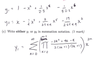# Forming a general summation of terms

• MathewsMD

#### MathewsMD

Hi,

I was trying to form a summation for ##y_1## and have provided a solution but do not quite understand how it was formulated. I was trying to look for general patters and besides a ##(-1)^{n+1}x^2n## in the numerator, I'm a little lost on how to find a general term for the denominator. Also, is the pi just another summation symbol inside of the sigma summation? Does the symbol have any other meaning? Any help regarding how to approach this questions would be greatly appreciated!

#### Attachments

•Screen Shot 2014-12-12 at 8.54.55 PM.png
13.6 KB · Views: 422
The $\prod$ symbol denotes a product instead of a sum.
For example
$\prod_{k=1}^3 (2k+ 1) = (2+1)(4+1)(6+1)$

Stephen Tashi said:
The $\prod$ symbol denotes a product instead of a sum.
For example
$\prod_{k=1}^3 (2k+ 1) = (2+1)(4+1)(6+1)$

Thank you. Just wondering, how was the summation formulated, though? I can check it and it works, but would not have devised that easily myself. Any hints on catching on to this particular pattern?

Were y1 and y2 just given to you? I notice they are handwritten. Did you derive them yourself?